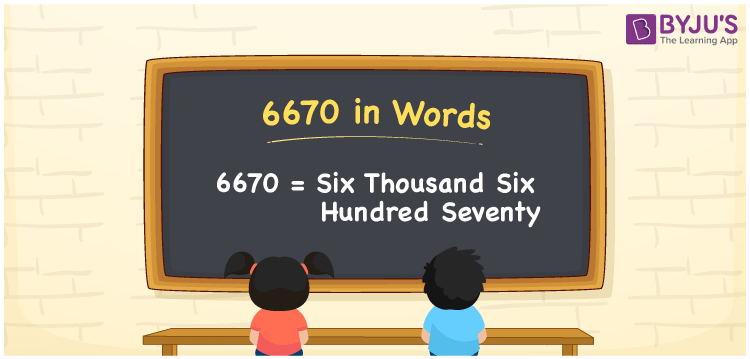# 6670 in Words

6670 in words is expressed as Six Thousand Six Hundred Seventy. For instance, Suman purchased a three burner gas stove worth Rs. 6670, then you can say, “Suman purchased a three burner gas stove worth Rupees Six Thousand Six Hundred Seventy”.6670 is a cardinal number since it denotes an exact quantity. This article explains the conversion of the number 6670 to word form with the help of simple tricks.

 6670 in Words Six Thousand Six Hundred Seventy Six Thousand Six Hundred Seventy in numerical form 6670

## 6670 in English Words

Generally, in Maths, we write the numbers in words using the letters of the English alphabet. Therefore, the number 6670 can be read in English as Six Thousand Six Hundred Seventy.## How to Write 6670 in Words?

We can convert the number 6670 in words using a place value chart. To create a place value chart for 6670, we need a table of 4 columns as it is a four-digit number. The place value chart for 6670 is given below.

 Thousands Hundreds Tens Ones 6 6 7 0

Hence, we can write the expanded form as:

6 x Thousand + 6 x Hundred + 7 x Ten + 0 x One

= 6 x 1000 + 6 x 100 + 7 x 10 + 0 x 1

= 6000 + 600 + 70 + 0

= 6000 + 600 + 70

= 6670

= Six Thousand Six Hundred Seventy

Hence, 6670 in words is written as Six Thousand Six Hundred Seventy

Interesting way of writing 6670 in words

6 = Six

66 = Sixty-Six

667 = Six Hundred Sixty-Seven

6670 = Six Thousand Six Hundred Seventy

Thus, the word form of the number 6670 is Six Thousand Six Hundred Seventy

6670 is a natural number that is the successor of 6669 and the predecessor of 6671

• 6670 in words – Six Thousand Six Hundred Seventy
• Is 6670 an odd number? – No
• Is 6670 an even number? – Yes
• Is 6670 a perfect square number? – No
• Is 6670 a perfect cube number? – No
• Is 6670 a prime number? – No
• Is 6670 a composite number? – Yes

## Frequently Asked Questions on 6670 in Words

Q1

### Write 6670 in words.

The number 6670 in words is Six Thousand Six Hundred Seventy.
Q2

### Simplify 6000 + 670, and express in words.

Simplifying 6000 + 670, we get 6670. The number 6670 in words is Six Thousand Six Hundred Seventy.
Q3

### 6670 is a composite number. True or False.

True, the number 6670 is a composite number.